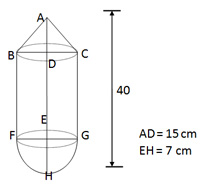# 3D Geometry: Mensuration- Sphere & Hemisphere

Understanding Three-Dimensional Geometry:
Most tests often include questions based on the knowledge of the geometries of 3-D objects such as cylinder, cone, cuboid, cube & sphere. The purpose of the
article is to help you learn basics of 3-D geometry and encapsulate some of the important formulae and tricks.
The questions on Volume and Surface Area appear in all the competitive exams. Most of the students tend to avoid this topic considering it to be quite complex and calculative. This article would help you not only in memorizing the formulas, but also in understanding direct or indirect applications of these formulas. We strongly advice you go through each and every definition and formula given below to solve questions on Surface Area and Volume.
Surface area and Volume Formulas
Solids: Solids are three–dimensional objects, bound by one or more surfaces. Plane surfaces of a solid are called its faces. The lines of intersection of adjacent faces are called edges.
For any regular solid, Number of faces + Number of vertices = Number of edges + 2. This formula is called Euler’s formula.
Volume: Volume of a solid figure is the amount of space enclosed by its bounding surfaces. Volume is measured in cubic units.
Sphere:  The set of all points in space, which are at a fixed distance from a fixed point, is called a Sphere. The fixed point is the centre of the sphere and the fixed distance is the radius of the sphere.
Volume = 4/3πr3. Surface Area (curved and total) = 4 πr2.
1. The radius of sphere is increased by 40 %. The increase in surface area of the sphere is:
1. 100%
2. 125%
3. 96%
4. 160%
2. A copper sphere of diameter 12 cm is drawn into a wire of diameter 8 mm. Find the length of the wire.
1. 19,440 cm
2. 1,800 cm
3. 30,520 cm
4. 48,600 cm
Hemisphere: A sphere cut by a plane passing through its centre forms two hemispheres. The upper surface of a hemisphere is a circular region.
Volume = 2/3πr3. Surface Area (curved) =2πr2. Surface Area (Total) = 2πr2 + πr2 (formula for area- plane) ⇒ 3πr2.
3. A cylinder and a hemisphere have equal volumes and equal bases. Find the ratio of height of cylinder to height of hemisphere.
1. 2:1
2. 3:1
3. 4:1
4. 5:1
5. 1:2
4. In the toy shown in the adjoining figure, the height of the cone = 15 cm, total height of the toy = 40 cm, radius of the hemisphere = 7 cm. Find the volume of the toy. (π = 22/7)
1. 1356.69 cm3
2. 4260.67 cmsup>3
3. 1065.16 cmsup>3
4. None of theseSpherical shell: If R and r are the outer and inner radius of a hollow sphere, then volume of material in a spherical shell = 4/3π (R3 – r3).
5. A solid hemisphere of radius 'a' that is made of a certain material weighs 60 kg. The figure is given below. What is the weight, in kg, of the entire hemispherical shell if the outer radius is '7a' and the inner radius is 3a?
1. 19888
2. 19860
3. 16890
4. 1,9080
5. 18960